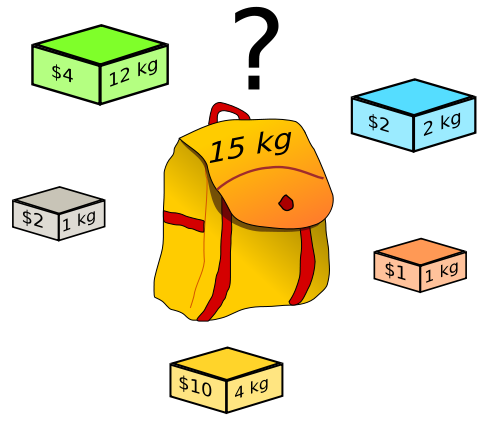# bzoj 2287 消失之物

2017成都联训Day2，身心疲惫~

# bzoj 2287 消失之物

## Description

ftiasch 有 N 个物品, 体积分别是 W1,W2,,WN$W_1, W_2, …, W_N$。 由于她的疏忽, 第 i 个物品丢失了。 “要使用剩下的 N – 1 物品装满容积为 x 的背包，有几种方法呢？” — 这是经典的问题了。她把答案记为 Counti,x$Count_{i,x}$ ，想要得到所有1iN,1xM$1 \leq i \leq N, 1 \leq x \leq M$Counti,x$Count_{i,x}$ 表格。## Sample Input

3 2
1 1 2

## Sample Output

11
11
21

# 来自黄学长的解题思路

Fi,j=1: j=0Fi1,j:j>wiFi1,j+Fi1,jwi:0<jwi

Counti,j=fn,j:0<j<wi$Count_{i,j}=f_{n,j} :0< j ：当物品i的体积大于j时，i本来就不可能被用到，自然与fn,j$f_{n,j}$相等。

Counti,j=fn,jCounti,jwi:jwi$Count_{i,j}=f_{n,j}-Count_{i,j-w_i}:j \geq w_i$：在这种情况下物品i会被使用，不用物品i填充j的体积的方案数就等于用所有物品填充体积j的方案数再减去其中使用了物品i的方案数。强制使用使用物品i填充j的体积，就相当于禁止使用物品i填充了jwi$j-w_i$的体积，（因为i占据了j中wi$w_i$的体积）。

# （不靠谱的）代码

#include<cstdio>
#include<cstdlib>
#include<algorithm>
using namespace std;
const int maxn=2000+10;
int w[maxn],f[maxn],c[maxn][maxn];
int main(){
int n,m;scanf("%d%d",&n,&m);f=1;
for(int i=1;i<=n;i++)scanf("%d",&w[i]);
for(int i=1;i<=n;i++){
for(int j=m;j>=w[i];j--){
f[j]=(f[j]+f[j-w[i]])%10;
}
}
for(int i=1;i<=n;i++){
c[i]=1;
for(int j=1;j<=m;j++){
if(j-w[i]>=0){
c[i][j]=(f[j]-c[i][j-w[i]]+10)%10;
}else c[i][j]=f[j];
printf("%d",c[i][j]);
}
printf("\n");
}
return 0;
}